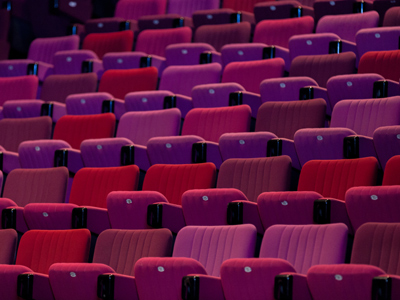The Royal Opera House has 2,256 seats. To the nearest 10, approximately how many seats are there?

# Level 5-6 Numbers - Approximating

In KS3 Maths you'll have to work out some problems involving large numbers. Before you work them out it's a good idea to have some idea of what the answer will be. That's where approximations come in.

Approximations give you a rough idea what the result of a calculation will be. Though you still have to work them out, the numbers involved are much easier to deal with as they end in zeros. Approximations are made by rounding numbers up or down to the nearest 10, 100 or even 1,000. Say you are asked to multiply 87 x 23. That would be hard but if you round the figures up/down it becomes simpler: 90 x 20 = 1,800. This answer is of course wrong, but it is quite close to the actual answer you want: 87 x 23 = 2,001. It is useful to approximate the answer to calculations so that you can check if your answers are correct.

Test your knowledge of approximating in the following quiz. Take your time and read each question carefully. Good luck!

1.
To the nearest 100, round 6,691 and 468.
6,000 and 400
6,000 and 500
6,600 and 500
6,700 and 500
I hope you didn't round 6,691 up to 7,000!
2.
By rounding 5,639 x 147 to the nearest 10, what is the approximate answer?
800,000
836,000
840,000
846,000
The calculation becomes 5,640 x 150 which equals 846,000
3.
By rounding to the nearest 10, what is the approximate answer to 1,537 / 222?
7
7.2
7.4
7.5
Remember to round the numbers in the calculation. 1,540 / 220 = 7
4.
To the nearest 100, round 4,483 and 4,999.
4,400 and 4,800
4,400 and 4,900
4,500 and 4,900
4,500 and 5,000
4,999 goes up 1 to 5,000 but 4,483 goes up 17 to 4,500
5.
By rounding to the nearest 10, what is the approximate answer to 79 x 22?
1,500
1,600
1,700
1,800
To approximate the answer, we need to round the numbers in the calculation. So 79 becomes 80 and 22 becomes 20. 80 x 20 = 1,600
6.
A tombola holds 27,600 tickets to the nearest 100. What is the maximum number of tickets in the tombola?
27,600
27,649
27,650
27,700
One more ticket and it would be rounded up to 27,700!
7.
To the nearest 10, round 4,483 and 4,999.
4,400 and 4,900
4,480 and 5,000
4,490 and 4,990
4,490 and 5,000
Be careful not to confuse 100s and 10s
8.
Give the answer to 7,743 / 5 to the nearest 100.
100
1,400
1,500
1,600
7,700 / 5 = 1,540 which is 1,500 to the nearest 100
9.
The Royal Opera House has 2,256 seats. To the nearest 10, approximately how many seats are there?
2,000
2,200
2,260
2,270
6 is closer to 10 than it is to 0
10.
The length of a football pitch is 110m to the nearest 10 metres. What is the smallest possible length it could be?
100
105
107
110
Remember to round up if the value is halfway!